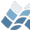NV5 GEOSPATIAL SOFTWARECHEBFIT

CHEBFIT

## Author

Craig B. Markwardt, NASA/GSFC Code 662, Greenbelt, MD 20770
craigm@lheamail.gsfc.nasa.gov
UPDATED VERSIONs can be found on my WEB PAGE:
http://cow.physics.wisc.edu/~craigm/idl/idl.html

## Purpose

Fit Chebyshev polynomial coefficients to a tabulated function

## Major Topics

Curve and Surface Fitting

## Calling Sequence

p = CHEBFIT(X, Y, ERR, INTERVAL=interval, NMAX=nmax,
PRECISION=prec, /EVEN, /ODD, REDUCE_ALGORITHM=)

## Description

CHEBFIT fits a series of Chebyshev polynomials to a set of
tabulated and possibly noisy data points. The functions MPFIT and
CHEBEVAL, available from the above web page, must also be in your
IDL path for this function to work properly. The user can choose
the desired precision and maximum number of chebyshev
coefficients.
This function is intended for use on already-tabulated data which
are potentially noisy. The user should never expect more than
NPOINTS terms, where NPOINTS is the number of (x,y) pairs. For
functions which can be evaluated to full machine precision at
arbitrary abcissae, the routine CHEBCOEF should be used instead.
For exact data tabulated on a regular grid, the routine CHEBGRID
should be tried.
The user can also specify that the function is even or odd, using
the keywords EVEN or ODD. This saves computation time because
certain terms in the expansion can be ignored. For the purposes
of this function even and odd refer to the symmetry about the
center of the interval.
The algorithm is employed in three steps. In the first step, the
coefficients are estimated at a crude level. In the second step,
it is determined whether certain coefficients are deemed
"ignoreable", i.e., they do not contribute significantly to the
function and are discarded. The operation of this step is
determined by the REDUCE_ALGORITHM keyword. Finally, the
remaining "good" coefficients are re-fitted to achieve the best
fit.

## Inputs

X, Y - the x- and y- tabulated values to be fitted.
ERR - (optional) the y-error bar associated with each (x,y) pair.
Default: 1

## Returns

An array of Chebyshev coefficients which can be passed to
CHEBEVAL. NOTE: the convention employed here is such that the
constant term in the expansion is P(0)*T0(x) (i.e., the convention
of Luke), and not P(0)/2 * T0(x).

## Keyword Parameters

EVEN, ODD - if set, then the fitting routine assumes the function
is even or odd, about the center of the interval.
INTERVAL - a 2-element vector describing the interval over which
the polynomial is to be evaluated.
Default: [-1, 1]
NMAX - a scalar, the maximum number of polynomial terms to be
fitted at one time.
Default: 16
PRECISION - a scalar, the requested precision in the fit. Any
terms which do not contribute significantly, as
defined by this threshold, are discarded. If the
function to be fitted is not well-behaved, then the
precision is not guaranteed to reach the desired
level.
Default: 1E-7
REDUCE_ALGORITHM - a scalar integer, describes how insignificant
terms are removed from the fit. If 0, then all terms
are kept, and none are dicarded. If 1, then only
trailing terms less than PRECISION are discarded. If
2, then both trailing and intermediate terms less than
Default: 2

## Example

x = dindgen(1000)/100 ; Range of 0 to 10
y = cos(x) + randomn(seed,1000)*0.01 ; Function with some noise
p = chebfit(x, y, interval=[0d,10d])
plot, x, y - chebeval(x,p, interval=[0d,10d])

## References

Abramowitz, M. & Stegun, I., 1965, *Handbook of Mathematical
Functions*, 1965, U.S. Government Printing Office, Washington,
D.C. (Applied Mathematical Series 55)
CERN, 1995, CERN Program Library, Function E407
Luke, Y. L., *The Special Functions and Their Approximations*,